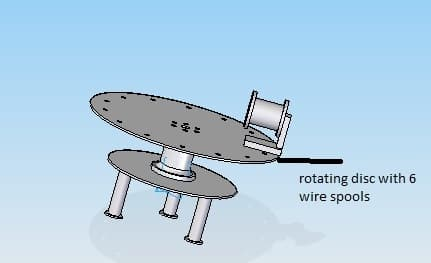CrazyEngineers Archive
Old, but evergreen and popular discussions on CrazyEngineers, presented to you in read-only mode.
@Nandan Rao • 23 Jul, 2014
hi everyone,
i need to rotate a horizontal disc which has bevel gear, the gear ratio of which is 1, the mass of disc along with its peripherals is around 33kg(MS disc)(M), the radius(r) of disc is 0.32m, i calculated the moment of inertia(I) by formula M(r)square/2 and then by angular acceleration formula (a/r)=(T/I), taking a=9.81m/s2...i found out the torque to be around 53Nm is the above procedure correct, and now if the calculation is correct should i look for a motor with 50Nm torque to drive the system?i need to rotate the upper disc i havent modeled the shaft in the 3d design shown,the mass mentioned before includes the mass of the spools, the lower disc is stationary so i havent considered it in my calculations..please help
@zaveri • 23 Jul, 2014 9.81 m/s2 is the value of acceleration due to gravity, and i don't think you can take that as default value for angular acceleration.

the angular acceleration value is the value which you desire it to be, and that is calculated using the value of the speed of rotation of the disc in RPM.

using the RPM, first calculate angular velocity using the formula (2*pi*RPM)/60.

then from this value of ang. velocity calculate the angular acceleration using the formula.
@zaveri • 23 Jul, 2014 The torque of the motor should always be greater than the torque value which you require.
@Nandan Rao • 24 Jul, 2014 taking RPM as 30 and time as 30sec, angular velocity was found to be 3.14rad/sec, and angular acceleration to be 0.104 rad/sec^2, and by the realtion of torque and angular acceleration, where, angular accln=(torque/Inertia) taking inertia of 1.78kg/m^2, by using this the torque was found to be 0.18Nm..is this right? should i consider FOS of 3 which will be 0.55Nm..and now my motor selection should be based on this torque??
@zaveri • 24 Jul, 2014 Yes.

Be very careful with the units while doing such calculations.
@Nandan Rao • 24 Jul, 2014 thank you sooo much<3!! 😀
@Dipak Kardani • 19 Dec, 2018

I have a disk of dia 760 mm and weight 25 kg and I need to rotate it through center axis with 300 RPM and acceleration time 2 sec what should my motor size with KW and Torque. ???? I calculated it is 45 NM torque and Power required to rotate disk with 300 RPM is 5.3 KW when take FOS = 2

5.5k views

## Related Posts

@NAVATHE SACHIN · Mar 24, 2013

please provide latest paper presentations for mechanical engineer.
4.7k views

@Kaustubh Katdare · May 24, 2015

The government of India has taken steps to setup 70 supercomputers to accelerate research work in various domains including weather and defence. These supercomputers will have varying speed of operations...
4.4k views

@neelam_neha · Aug 6, 2008

i wil gve post on this topic later but first i want a suggestion frm all of u......
3.9k views

@CIVILPRINCESS · Jan 24, 2011

Hiya CEans 😁 here is one simple quiz for you. 😀 i had set these for a club activity in my college. now you guys try to answer these questions...
3.6k views

@jGOAT · Jun 28, 2012

Hi,I recently completed my Bachelors degree.(June 2012)B.Tech in Computer Science.Up until the second year of my engineering, I was a below average student.But, afterwards, I started working hard and my...
3.5k views